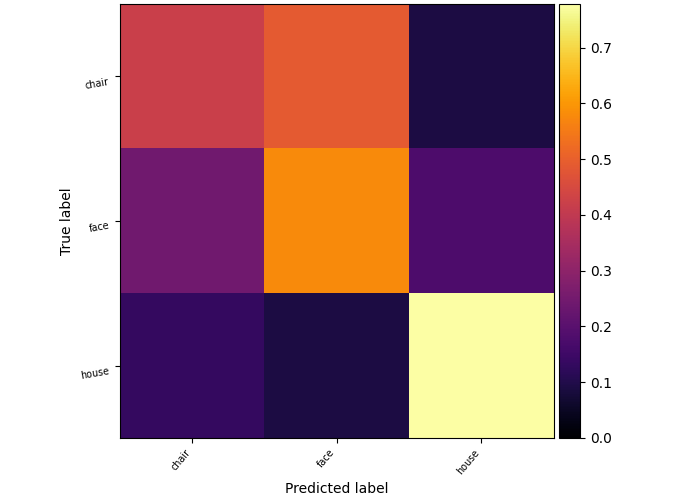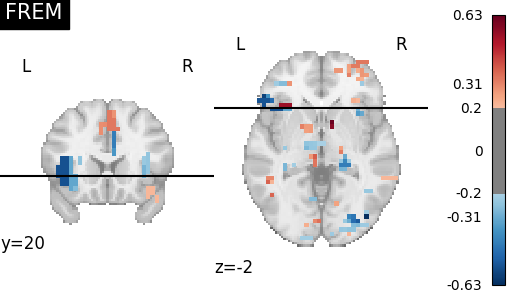# 2.3. FREM: fast ensembling of regularized models for robust decoding¶

FREM uses an implicit spatial regularization through fast clustering and aggregates a high number of estimators trained on various splits of the training set, thus returning a very robust decoder at a lower computational cost than other spatially regularized methods. Its performance compared to usual classifiers was studied on several datasets in [Hoyos-Idrobo et al. 1].

## 2.3.1. FREM pipeline¶

FREM pipeline averages the coefficients of many models, each trained on a different split of the training data. For each split:

• aggregate similar voxels together to reduce the number of features (and the computational complexity of the decoding problem). ReNA algorithm is used at this step, usually to reduce by a 10 factor the number of voxels.

• optional : apply feature selection, an univariate statistical test on clusters to keep only the ones most informative to predict variable of interest and further lower the problem complexity.

• find the best hyper-parameter and memorize the coefficients of this model

Then this ensemble model is used for prediction, usually yielding better and more stable predictions than a unique model at no extra-cost. Also, the resulting coefficient maps obtained tend to be more structured.

There are two object to apply FREM in Nilearn:

They can use different type of models (l2-SVM, l1-SVM, Logistic, Ridge) through the parameter ‘estimator’.

## 2.3.2. Empirical comparisons¶

### 2.3.2.1. Decoding performance increase on Haxby dataset¶In this example we showcase the use of FREM and the performance increase that it brings on this problem.

Code

The complete script can be found here.

### 2.3.2.2. Spatial regularization of decoding maps on mixed gambles study¶Code

The complete script can be found here.